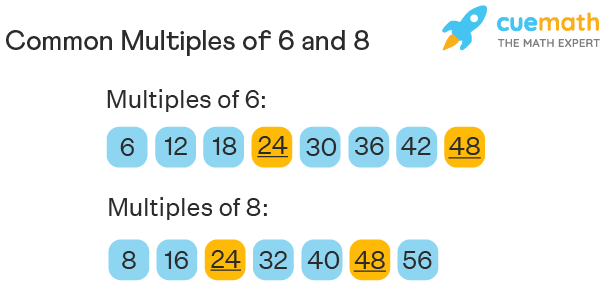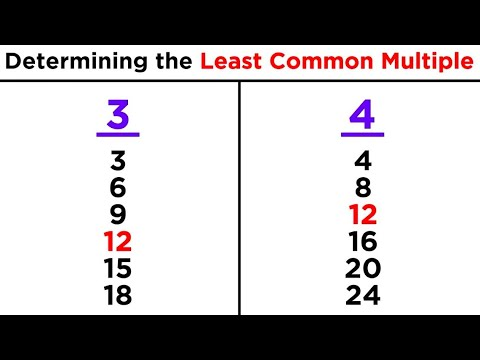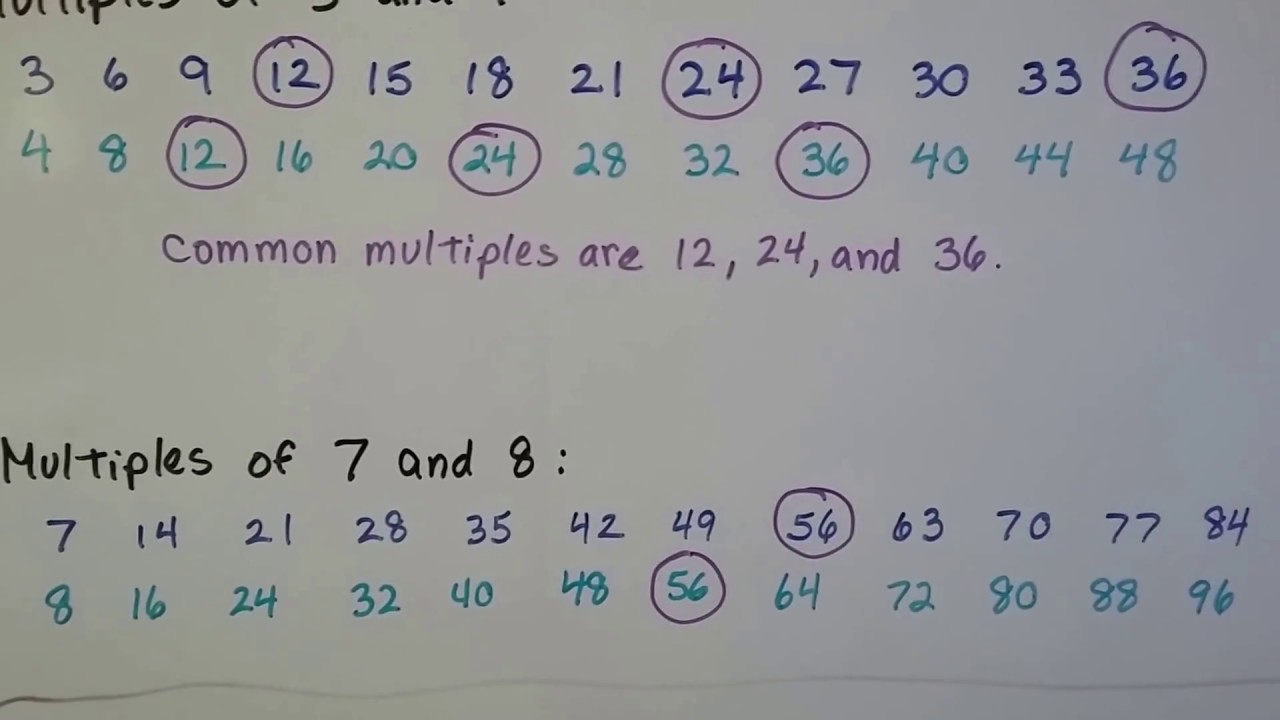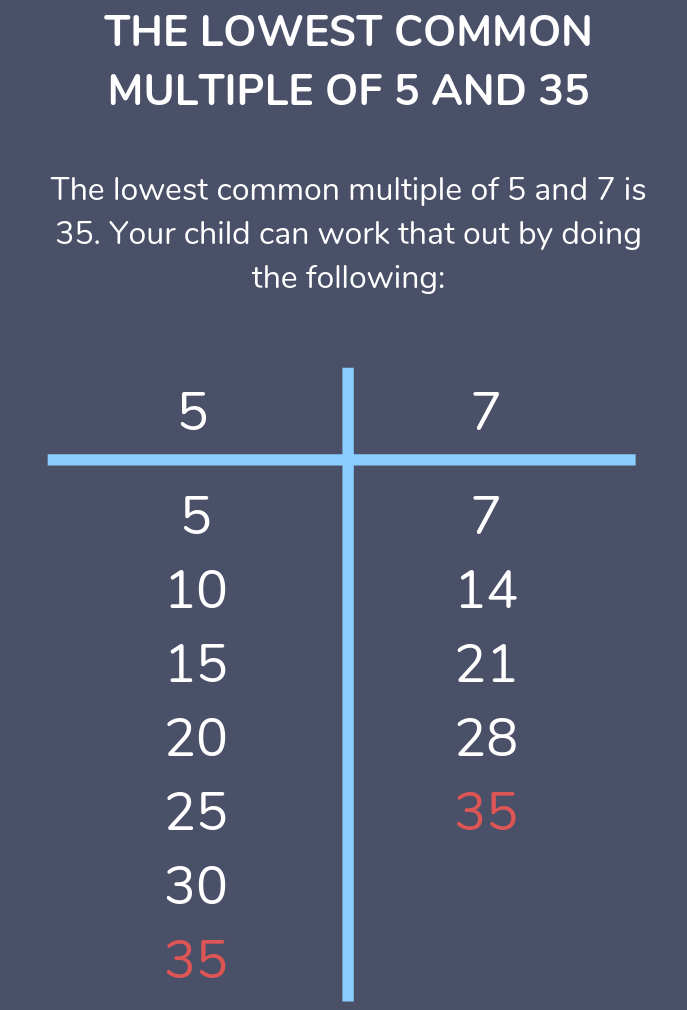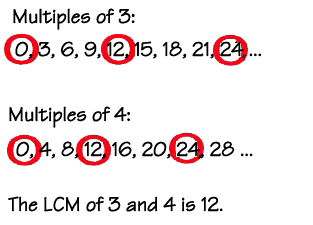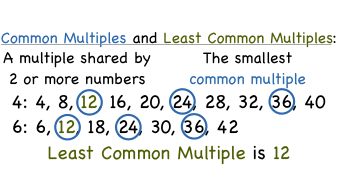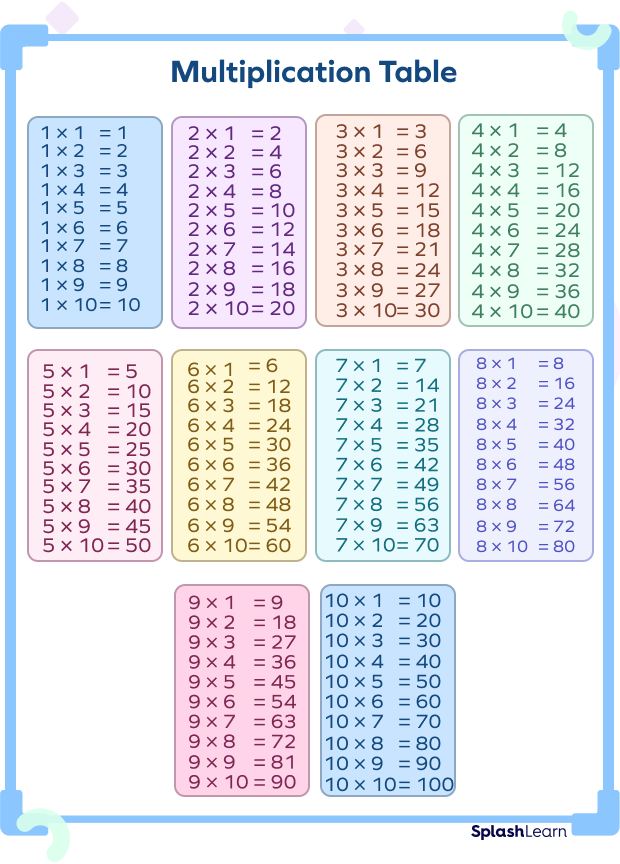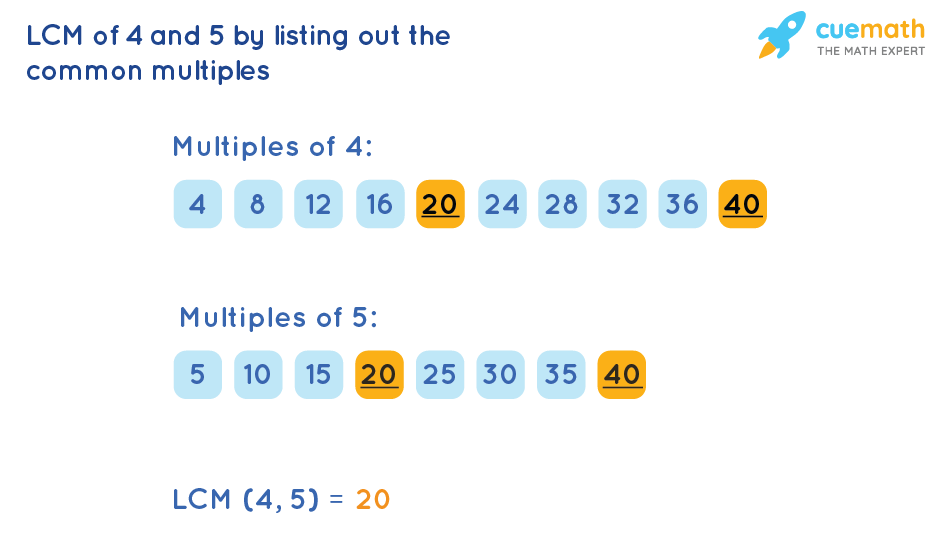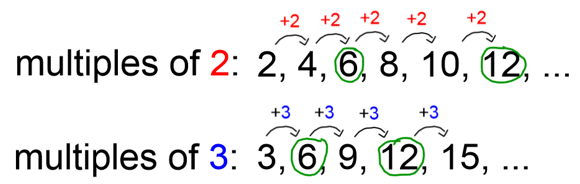# History of Common Multiples:

##### The History of Common Multiples:

The concept of multiples can be traced back to ancient civilizations, where people used counting and arithmetic for practical purposes such as trade and agriculture. The idea of finding common multiples emerged later, as people began to solve more complex problems involving multiple quantities.

 Q: What is a common multiple? A: A common multiple is a number that is a multiple of two or more numbers. Q: Why are common multiples important? A: Common multiples are important because they help us compare and manipulate different quantities, leading to more efficient problem-solving and better understanding of mathematical concepts. Q: How do you find common multiples? A: To find common multiples, you need to list the multiples of each number and identify the ones that appear in both lists. Alternatively, you can use prime factorization to find the least common multiple (LCM). Q: What are some examples of common multiples? A: Examples of common multiples include 6, 12, 18, and 24, which are common multiples of 2 and 3.
##### Conclusion:

In conclusion, common multiples are a fundamental concept in mathematics, helping us compare and manipulate different quantities. From ancient civilizations to modern-day science and engineering, common multiples have played a crucial role in diverse fields where multiple variables need to be analyzed and optimized. By understanding and mastering the concept of common multiples, we can develop stronger problem-solving skills and gain a deeper appreciation for the fascinating world of mathematics.

##### Timeline of Common Multiples:
 Ancient civilizations: People used counting and arithmetic for practical purposes. Middle Ages: Algebra and other advanced mathematical concepts began to emerge. Modern era: The study of multiples became a crucial component of mathematics, contributing to diverse fields such as engineering, physics, and computer science.
##### Interesting Facts about Common Multiples:
 The concept of common multiples is closely related to the idea of divisibility, which involves determining whether one number can be divided by another without leaving a remainder. Common multiples play a crucial role in diverse fields such as engineering, physics, and computer science, where they are used to analyze and optimize systems with multiple variables. The least common multiple (LCM) is the smallest positive integer that is a multiple of two or more numbers, making it a useful tool in many mathematical applications.
##### Image Gallery: